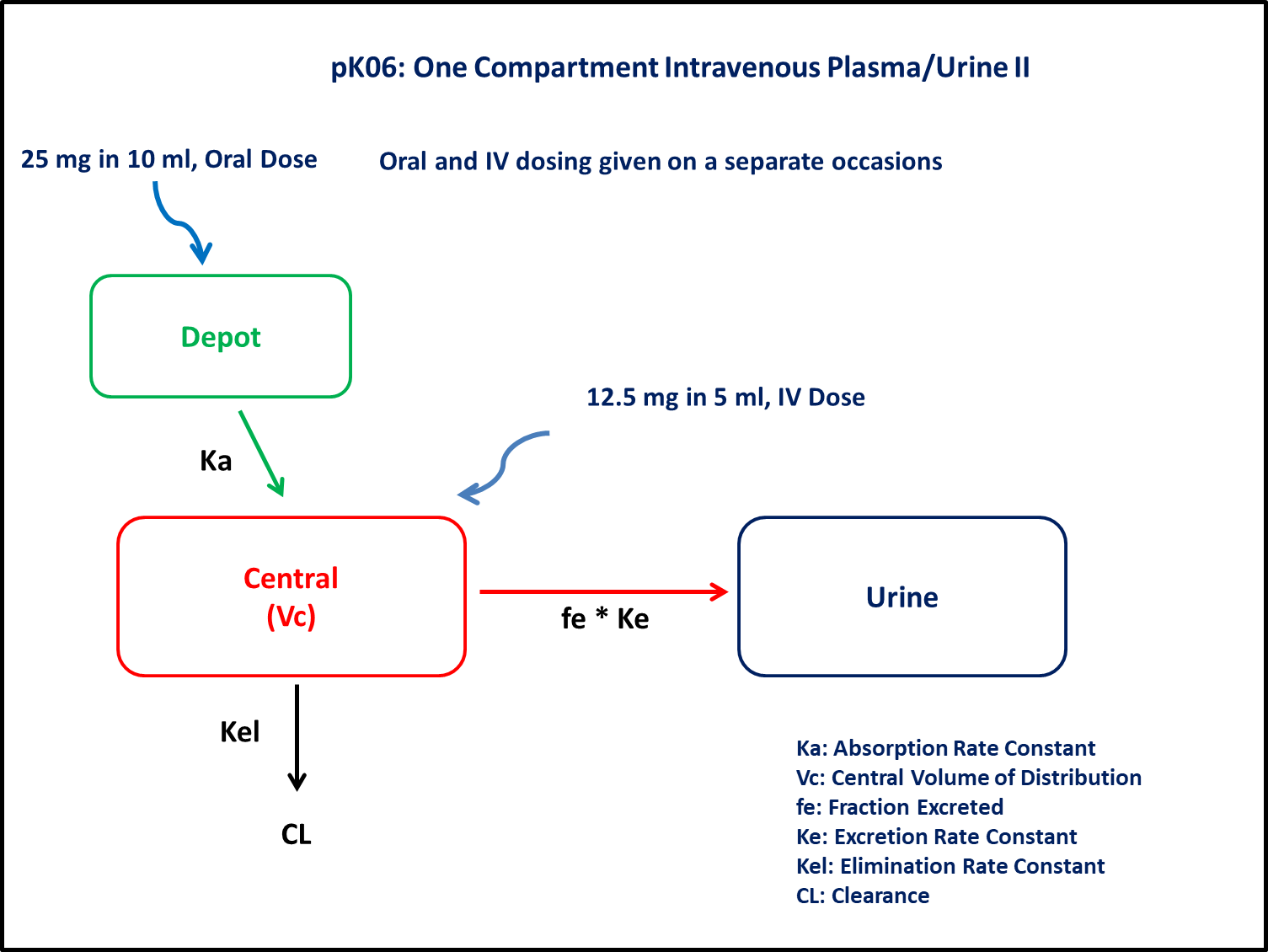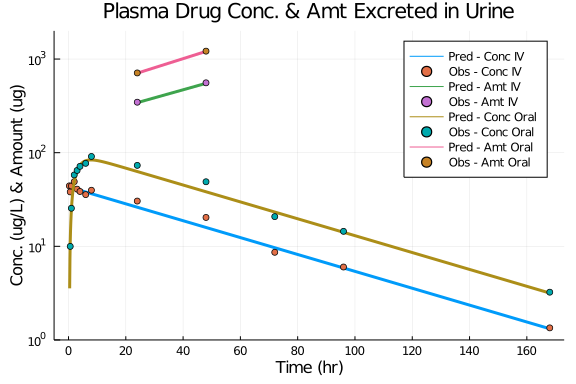# Exercise PK06 - One Compartment Intravenous Plasma/Urine II

### Background

• Structural model - One compartment linear elimination with first order absorption

• Route of administration - Oral and IV given simulataneously

• Dosage Regimen - 100 mg IV and 100 mg Oral

• Number of Subjects - 1### Objective

In this tutorial, you will learn to build one compartment model and to simulate the model for different subjects and dosage regimens.

### Libraries

call the "necessary" libraries to get started

using Pumas
using Plots
using CSV
using StatsPlots
using Random


### Model

In this one compartment model, we administer dose both oral and iv at two different occasions.

pk_06        = @model begin
@param begin
tvcl     ∈ RealDomain(lower=0)
tvvc     ∈ RealDomain(lower=0)
tvfe     ∈ RealDomain(lower=0)
tvka     ∈ RealDomain(lower=0)
tvlag    ∈ RealDomain(lower=0)
tvF      ∈ RealDomain(lower=0)
Ω        ∈ PDiagDomain(6)
σ²_prop  ∈ RealDomain(lower=0)
end

@random begin
η        ~ MvNormal(Ω)
end

@pre begin
Cl       = tvcl * exp(η)
Vc       = tvvc * exp(η)
Ka       = tvka * exp(η)
fe       = tvfe * exp(η)
lags     = (Depot = tvlag * exp(η),)
bioav    = (Depot = tvF * exp(η),)
end

@dynamics begin
Depot'   = -Ka*Depot
Central' =  Ka*Depot - (Cl/Vc)*Central
Urine'   =  fe*(Cl/Vc)*Central
end

@derived begin
cp       = @. (Central/Vc)
dv       ~ @. Normal(cp, sqrt(cp^2*σ²_prop))
ae       = @. Urine
end
end

PumasModel
Parameters: tvcl, tvvc, tvfe, tvka, tvlag, tvF, Ω, σ²_prop, σ_add
Random effects: η
Covariates:
Dynamical variables: Depot, Central, Urine
Derived: cp, dv, ae, dv_ae
Observed: cp, dv, ae, dv_ae


### Parameters

Parameters provided for simulation. tv represents the typical value for parameters.

• Cl - Clearance (L/hr)

• Vc - Volume of Central Compartment (L)

• Fe - Fraction of drug excreted in Urine

• lag - lag time (hr)

• Ka - Absorption rate constant (hr⁻¹)

• F - Bioavailability

• Ω - Between Subject Variability

• σ - Residual error

param = ( tvcl    = 6.02527,
tvvc    = 290.292,
tvfe    = 0.0698294,
tvka    = 0.420432,
tvlag   = 0.311831,
tvF     = 1.13591,
Ω       = Diagonal([0.0,0.0,0.0,0.0,0.0,0.0]),
σ²_prop = 0.015,

(tvcl = 6.02527, tvvc = 290.292, tvfe = 0.0698294, tvka = 0.420432, tvlag =
0.311831, tvF = 1.13591, Ω = [0.0 0.0 … 0.0 0.0; 0.0 0.0 … 0.0 0.0; … ; 0.
0 0.0 … 0.0 0.0; 0.0 0.0 … 0.0 0.0], σ²_prop = 0.015, σ_add = 3)


### Dosage Regimen

##### For IV

A single subject receives an IV-bolus dose of 12.5 mg or 12500μg

ev1  = DosageRegimen(12500, time=0, cmt=2)
sub1 = Subject(id=1, events=ev1)

Subject
ID: 1
Events: 1

##### For Oral

A single subject receives an oral dose of 25 mg or 25000μg

ev2  = DosageRegimen(25000, time=0, cmt=1)
sub2 = Subject(id=2,events=ev2)

Subject
ID: 2
Events: 1


### Simulation

Here, we will learn how to simulataneously simulate plasma conc and urine amount after IV and Oral administration.

##### For IV

Lets simulate for plasma concentration and amount excreted unchanged in urine.

Random.seed!(123)
sim_sub1_iv = simobs(pk_06, sub1, param, obstimes=0.1:0.1:168)
df_sim_iv   = DataFrame(sim_sub1_iv)

##### For Oral

Lets simulate the plasma concentration and amount excreted unchanged in urine.

Random.seed!(123)
sim_sub2_oral = simobs(pk_06, sub2, param, obstimes=0.1:0.1:168)
df_sim_oral   = DataFrame(sim_sub2_oral)
filter!(x -> x.time .> 0.2, df_sim_oral)


### Dataframe & Plot

Combined Plot of IV data and Oral data

df_iv_plasma   = filter(x -> x.time in [0.3, 0.6, 1, 2, 3, 4, 6, 8, 24, 48, 72, 96, 168], df_sim_iv)
df_iv_urine    = filter(x -> x.time in [24,48], df_sim_iv)
df_oral_plasma = filter(x -> x.time in [0.3, 0.6, 1, 2, 3, 4, 6, 8, 24, 48, 72, 96, 168], df_sim_oral)
df_oral_urine  = filter(x -> x.time in [24,48], df_sim_oral)

@df df_sim_iv plot(:time,:cp, yaxis=:log,
title="Plasma Drug Conc. & Amt Excreted in Urine",label="Pred - Conc IV",
xlabel="Time (hr)", ylabel="Conc. (ug/L) & Amount (ug)", linewidth=3,
xticks=[0,20,40,60,80,100,120,140,160,180], ylims=(1,2000))
@df df_iv_plasma scatter!(:time, :dv, label="Obs - Conc IV")
@df df_iv_urine plot!(:time, :ae, label="Pred - Amt IV", linewidth=3)
@df df_iv_urine scatter!(:time, :dv_ae, label="Obs - Amt IV")
@df df_sim_oral plot!(:time,:cp, yaxis=:log, label="Pred - Conc Oral", linewidth=3)
@df df_oral_plasma scatter!(:time, :dv, label="Obs - Conc Oral")
@df df_oral_urine plot!(:time, :ae, label="Pred - Amt Oral", linewidth=3)
@df df_oral_urine scatter!(:time, :dv_ae, label="Obs - Amt Oral", linewidth=3)